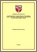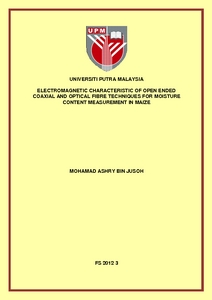Simple Search:

# Electromagnetic characteristic of open ended coaxial and optical fibre techniques for moisture content measurement in maize

## Citation

Jusoh, Mohamad Ashry (2012) Electromagnetic characteristic of open ended coaxial and optical fibre techniques for moisture content measurement in maize. PhD thesis, Universiti Putra Malaysia.

## Abstract / Synopsis

This thesis describes an electromagnetic characteristic of open ended coaxial and optical fibre techniques for moisture content measurement in maize kernel based on reflectivity measurements. Both analytical and numerical methods were employed to calculate the reflectivity of the sensors. The former utilized the admittance model whilst the latter was implemented using Finite Element Method (FEM). The admittance model is an analytical equation based on integral equation for electric field at aperture. Computation of reflectivity using FEM is based on the solutions of a set of partial differential electric field equations. The FEM modelling was implemented using COMSOL Multiphysics version 3.5 software. Permittivity values required as inputs to admittance and FEM that were obtained from dielectric mixture model. The measurement setups for open ended coaxial technique consist of Agilent Professional Network Analyzer (PNA-L N5230A) in microwave frequency range between 1 GHz and 5 GHz. The calibration was performed using a full one-port calibration technique. The optical fibre technique reflectivity measurement was realized using Ocean Optic Spectrometer (USB4000) with an operating wavelength between 230 nm and 800 nm. In this work, the open ended coaxial technique involves both magnitude and phase whilst the optical fibre only provides the reflectivity value (magnitude only) The FEM results showed better agreement with measured data than the admittance model for both sensors. The effect of mesh was studied thoroughly and was found that the maximum relative errors for FEM with 4454 number of elements were 0.0093 and 0.0861 for magnitude and phase respectively. Calibration equations have been established to determine moisture content in maize based on reflectivity measurements using both open ended and optical fibre sensors. The actual moisture content was found from standard oven drying method. The mean percentage errors of the calibration equations were found to be less than 4.32 % and 4.02 % for magnitude and phase of reflection coefficient respectively. While for optical fibre sensor, it was found that the mean percentage error are 12.23 % and 9.16 % for 300 nm and 800 nm respectively. The empirical models have been developed to predict permittivity of maize based on measured reflection coefficient of sensors. The mean percentage errors were found to be less than 10 % when compared to commercial values. Enhanced accuracy of prediction of permittivity was obtained by introducing an objective function to determine unknown parameters of the mixture model.Preview
PDF
FS 2012 3R.pdfView Item